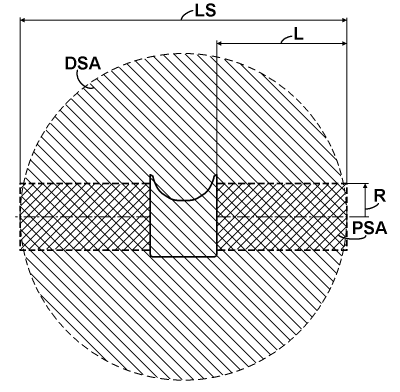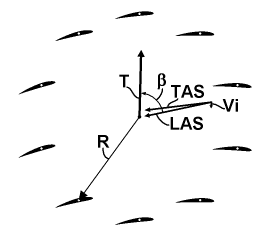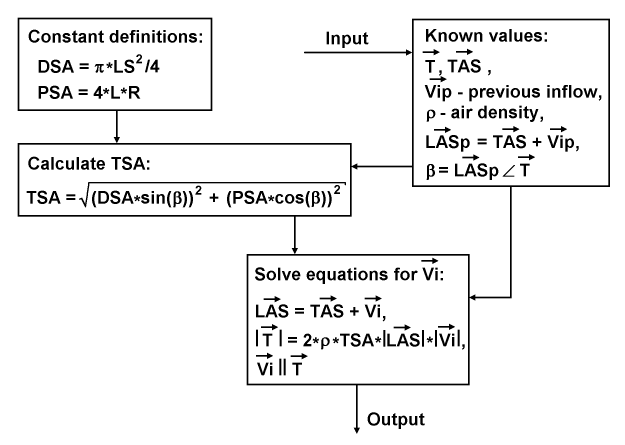﻿ Liftoplane: Inflow

# Inflow

Next aerodynamic aspect is related with the induced drag. It is routine practice for airplanes using special formulas for calculate the induced drag and related correction of the lift for a given aspect ratio. Those formulas reflect changes in the drag and the lift created by influence of an inflow, which depends from the lift distribution over the wings. But the practice isn't applicable for modeling powered actuators like the presented rotor or the rotor of a helicopter, because the modeling implies to know a particular lift and a drag of each particular wing of the rotor. And simple application of mentioned formulas on each separated wing isn't correct, due to mutual influences of wings. This problem can be simple resolved upon knowing the inflow itself. And so the next aerodynamic aspect for the modeling of presented rotor is calculation of the entire inflow.

The inflow has a simple relation with the thrust specific area (TSA) of an actuator. From the lifting line theory and from the point of view of the momentum theory of actuators it is known: for a monoplane with the elliptically loaded wings toward the wingspan direction, the TSA is simple the area of a circle based on the wingspan diameter. But presented rotor isn't having the elliptical load distribution. It has presumable the equal load distribution. This kind of distribution also very useable for a monoplane modeling and it implies some coefficient of efficiency, reflected an additional increasing of the induced drag due to the non-constant distribution of the induced speed, which is practically above 0.85. But the presented rotor from the glider's point of view isn't a monoplane. For much of flight operations it can be substituted as a tetra-plane with the average wing separation about 0.05 to 0.07 of the wingspan or as a bit under-performing triplane with the average wing separation about 0.075 to 0.1 of the wingspan. From the work of L. Prandtl "Induced drag of multiplanes", published in NACA TN 182, 1924, a coefficient of the induced drag of that triplane over an equivalent monoplane can be simple found. It lays between 0.852 and 0.824 for the referenced wing separation range respectively. This decreasing of the induced drag will overlap that increasing due to the non-elliptical load. So, for having a pessimistic appreciation it can be assumed: the induced drag of the presented rotor is equal to the induced drag of the elliptically loaded equivalent monoplane. And so, going from the induced drag to the inflow, TSA of the presented rotor in the case of gliding will be the area based on its wingspan LS, as it is presented on the chart below.I reference the area as the downwash specific area (DSA), since it has a direct logical connection with the downwash feature. An impact of the projection cross-area of the fuselage will a bit decrease the DSA, but from other side it will a bit increase the wings separation of the equivalent triplane, so I neglected this impact. The presented rotor exhibits also other kind of TSA: it is the propulsion specific area (PSA), which is actualizing upon beginning of the acceleration on the runway. I selected the area as the sum of cross-areas of the two cylinders based on both rotors with the radius R equal to the distance of the pivot axes of the wings from the center of rotors and the length L for each.

Other parameter need for the calculation of inflow is the trust specific angle, which is simple referenced as β. The chart below explains its relation with the thrust and the airspeed.The inflow moderates the TAS to the common LAS of the rotor. And that LAS has some angle relative to the thrust vector T. It is the β angle. Its impact on the inflow is follows: when the β equal to zero, the rotor performs as a pure propeller with the TSA equal to the PSA, and when the β equal to 90°, the rotors performs as a pure glider with the TSA equal to the DSA. In any intermediate case the TSA can be calculated by a simple quadrature formula based on the both orthogonal components and the angle β. It is interesting, for a helicopter those both areas PSA and DSA are equal, when the PSA is considered for hovering, so practically a helicopter has a constant TSA.The diagram above represents a data flow and definition for the calculation of the inflow. At first, there are constant definitions for DSA and PSA. At second, there is an input of known value, including the thrust vector T, the TAS vector, a vector of the previous inflow Vip, the air density ρ and also chain calculated values of the previous LAS vector LASp and the β angle. The process continues in calculation TSA with the quadrature formula, which utilizes the DSA, the PSA and the β. The resulted TSA enters together with the TAS and the thrust vectors into a routine for solving equations based on the desired inflow vector Vi. These equations consist from: the equation for a presumed total flow LAS; the scalar equation reflected the moment conservation law based on the presumed total mass flow and magnitude of the unknown inflow; and the relation for restoring entire inflow vector. The routine resolves two first equations together by using the iterative Newton method and restores the entire inflow vector to the output.